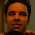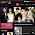## Monday, 26 December 2011

### Modelling returns using PCA : Evidence from Indian equity market

As my finance term paper, I investigated an interesting question where I tried to identify macroeconomic variables that explain the returns on equities. Much of the debate has already taken place on this topic which has given rise to two competing theories of asset pricing viz. CAPM (capital asset pricing theory or single factor model) and APT (arbitrage pricing theory or multi-factor model). Here is a brief discussion on the two in my previous post. In this post I would like to discuss my approach to answering this question in the context of Indian stock market.

Methodology:
• Companies that have been actively traded on NSE stock exchange for the past 10 years (218 companies) were selected and their daily stock returns data for these 10 years was taken from PROWESS.
• Using PCA, first 10 components from the returns data of the 218 companies was extracted. More on PCA in my previous post, here
• These components were then separately regressed first on NIFTY returns (first regression)
• Then these components were regressed on NIFTY returns, MIBOR rate changes, and INR/USD exchange rate changes (second regression).
• The explanatory power of the 2 regressions were compared using a F-statistic. (refer to pg. 10 in the paper attached in the end of the post)

Findings and R codes:
We start with calculating the PCA of the returns on the 218 companies daily return data, then employing the 2 regressions, then comparing the 2 regressions using a F-statistic. F-stat tells us if there is any additional explanation offered when we include macroeconomic variables (viz. MIBOR, INR/USD) in our equation.

The results that I obtained pose an interesting observation. We find that the F-stat is significant at 5% for 7 out of the 10 regressions, meaning that out of the 10 regressions (each regression with a separate component) we find statistically significant addition in the explanatory power of the model after adding the macroeconomic variables. Therefore, on statistical ground I can argue that a multi factor model (APT) is preferable over a single factor model (CAPM) for modelling stock returns in the case of Indian equity market. This assertion, if holds true, can have reaching implications for asset pricing for Indian securities. Let me explain why. The principal components (that are the dependent variables in the model) are essentially the common factor across all the companies stock returns with the idiosyncratic effects discounted, so any variables that explains this common component would be the systematic risk (think why!). Now we can relate it to the debate between the CAPM and APT guys. If the CAPM guys were correct, I would obtain no additional explanation in my model after adding the macroeconomic variables i.e their assertion that the market risk (market beta) capture the entire systematic risk holds true.

The results, however, suggest that in 7 out of 10 regressions there is statistically additional explanation offered by the macroeconomic variables. Well, so we can out-rightly reject the applicability (of the much prevalent) CAPM in the case of Indian equities. Or is there something amiss? Now if I closely look at the absolute increase in the explanatory power by looking at the Adjusted-R-squared values before and after the addition of the macroeconomic variables, the absolute increase in all the cases is < 1% (refer to pg. 11 in the paper at the end of this post). Therefore, although we obtain statistical efficiency after the addition of the variables, the economic efficiency (intuition) is called to question. Is it worth while to complicate our model with additional macroeconomic variables, when we can simply have the market rate used as a reasonable proxy for all the variables? And all this just to prove a point that we have macro-variables that can provide 0.5% additional explanation in our model? This takes us back to the eternal debate of statistical vs economic efficiency, what is more important? Is the above result robust enough (on economic intuition) to question the much used, simple and powerful CAPM? Is there a threshold even in statistical efficiency to ensure economic efficiency? These are some questions that still linger on in my mind.

If we view the above result with this caveat of economic efficiency then there is reason for us to believe that a single factor model would be a preferable way to model stock returns. There are, however, evidences in the literature to suggest that multi factor (APT) is a superior way of modelling returns, but the identification of these "multi factors" remains a contentious issue among the researchers. In some desperate attempts to refute CAPM, researcher extracted principal components from a number of macroeconomic variables as the input to the PCA. This resulted in factors that had no economic intuition at all, that were then used as independent variables in explaining the returns. The APT (Arbitrage pricing theory) is a 'theory', whereas CAPM is a 'model' that approximates reality. So even if in reality there are multiple factors that give rise to the returns signals as we see them, the identification of these factors is not a trivial exercise as we have seen above. Statistically we managed to overturn the CAPM in the context of Indian equity markets but in term of economic intuition the results do not seem to be that promising. Therefore, the above exercise tells us exactly why people still stick to the evergreen CAPM as an asset pricing model.

In case you wish to replicate the exercise the data can be obtained from here: Returns_CNX_500Nifty_returnsMIBORExchange_rates.

Here is the full text of my paper. Feedback are welcome.

1.Hi
That is very nice work, yet I think it is already well established that the CAPM is not enough to explain market move, it was what you called "overturned" by fama frnech (1973). Not to mitigate the contribution of seeing how it is done in practice.

2.Hi eran,

Thank you for your comment. Indeed it is well established that CAPM is not enough to explain stock returns, but to identify the factors (or variables) that offer this explanation has been an eternal debate among researcher. The findings in my paper add to this debate. Although my results establish the inferiority of CAPM statistically, but the quantum of addition in the explanatory power by my multi factor model is minuscule.

Now the answer to the question "Is it worthwhile to add the macro-variables in the model?" deserves a more critical scrutiny than just rejoicing at a F-statistic that is significant at 5%.

~
Shreyes

3.Hmm.. nice to see somebody I know using lambda functions. But why the mixing with imperative style?

4.Hey Akhil,

PCA is essentially done using eigen value decomposition (EVD) of the var-covar matrix of the input data. I am not aware if there is any lambda function involved in the computation, but since I have just used PCA as I tool in answering an economic question, my understanding on PCA is limited.

Since the computation part was easily taken care of by R (simple command princomp()) I dint bother looking up what happens behind the codes. Would really like to know what you were referring to when you said lambda function and the imperative style.

5.My guess is that Akhil S Behl's term "lambda functions" was referring to your use of what in R are often called "anonymous functions". It is a reference to the "lambda calculus" often attributed to Church IIRC. Your command : cfs1 <- sapply(reg.apt, function(x) as.vector(x\$coefficients)) might be rewritten in imperative stye as: for (i in seq_along(reg.apt)){cfs1[i] <- as.vector( reg.apt[i][["coefficients"]])

6.Thank you for you comment.

I wasn't aware they were called lambda function. And the mix that you see here in the lambda function and imperative style is because the codes were created with the help of Utkarsh (my pro bro). So needless to say the imperative (and straight forward) style is my contribution and the rest was courtesy Pro bro ;-)

~
Shreyes

7.Information
Informatics Advanced World collects and shares highly scalable Informatics Data and organizes it for good interpretation, simple and understandable presentation so that any one can easily analyze and spread such Information.

8.Hello,

Thank you for you article, is it possible for you to share your code?

Best regards

9.Modeling not only the outer beauty, it helps you exploring your inner beauty by discovering your ambitions........ Top Modeling Agency in India and Best Modeling Agency In India